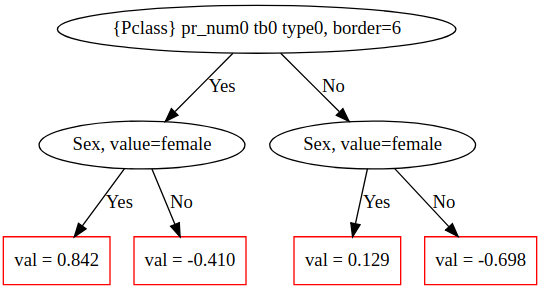# plot_tree

Visualize the CatBoost decision trees.
Note. This method is suitable only for the set by default SymmetricTree tree growing policy (set in the grow_policy parameter).

graphviz

## Method call format

``plot_tree(tree_idx, pool=None)``

## Parameters

Parameter Possible types Description Default value
tree_idx int

The index of the tree from the model that should be visualized.

Required parameter
pool catboost.Pool

An optional parameter for models that contain only float features. Allows to pass a pool and label features with their external indices from this pool. If the pool is not input, internal indices are used.

For example, for a semicolon-separated pool with 2 features “f1;label;f2” the external feature indices are 0 and 2, while the internal indices are 0 and 1 respectively.

None

Required for models with one-hot encoded categorical feature

Parameter Possible types Description Default value
tree_idx int

The index of the tree from the model that should be visualized.

Required parameter
pool catboost.Pool

An optional parameter for models that contain only float features. Allows to pass a pool and label features with their external indices from this pool. If the pool is not input, internal indices are used.

For example, for a semicolon-separated pool with 2 features “f1;label;f2” the external feature indices are 0 and 2, while the internal indices are 0 and 1 respectively.

None

Required for models with one-hot encoded categorical feature

## Type of return value

graphviz.dot.Digraph

## Usage examples

``````import numpy as np
import catboost
from catboost import CatBoostClassifier, Pool

from catboost.datasets import titanic
titanic_df = titanic()

X = titanic_df.drop('Survived',axis=1)
y = titanic_df.Survived

is_cat = (X.dtypes != float)
for feature, feat_is_cat in is_cat.to_dict().items():
if feat_is_cat:
X[feature].fillna("NAN", inplace=True)

cat_features_index = np.where(is_cat)
pool = Pool(X, y, cat_features=cat_features_index, feature_names=list(X.columns))

model = CatBoostClassifier(
max_depth=2, verbose=False, max_ctr_complexity=1, iterations=2).fit(pool)

model.plot_tree(
tree_idx=0,
pool=pool
)``````

An example of a plotted tree:## Tutorial

Refer to the Visualization of CatBoost decision trees tutorial for details.